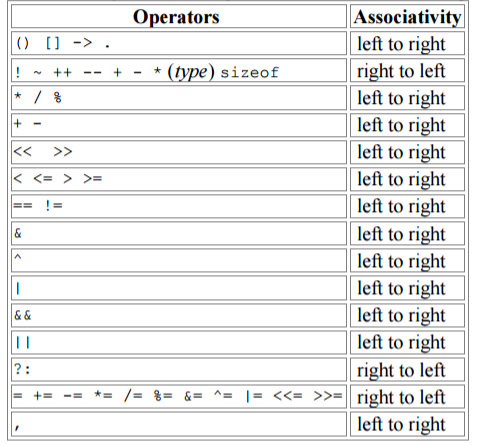# Difference between i++ and ++i

Date Tags c

I'm starting to read through Hacking: The Art of Exploitation on my four hours daily commute to work in order to get myself more comfortable working with C. I resolve a puzzle I have about C, as shown in the title.

• i++ means increment the value of i by 1 after evaluating the arithmetic operation.
```int a, b;
a = 5;
b = a++ * 6;
```

b will contain 30 and a will contain 6, since the shorthand of b = a++ * 6; is the equivalent to the following statements:

```b = a * 6;
a = a + 1;
```
• ++i means increment the value of i by 1 before evaluating the arithmetic operation.
```int a, b;
a = 5;
b = ++a * 6;
```

b will contain 36 and a wil contain 6, since the shorthand of b = ++a * 6; is the equivalent to the following statement:

```a = a + 1;
b = a * 6;
```

In fact, the two principles mentioned above apply more than "evaluating the arithmetic operation". For example, in the stack push operation:

```/* Push an element on the Stack
*/
void
push (ET elem, Stack S)
{
if (isFull(S))
{
resizeStack(S);
}
S->Array[++S->TopOfStack] = elem;
}
```

we can do S->Array[++S->TopOfStack] = elem;, which is equivalent with the following, a nice short verison:

```S->Array[S->TopOfStack] = elem;
S->TopOfStack = S->TopOfStack + 1;
```

Another example is stack topAndPop:

```/* Check the top element and pop it out of Stack
*/
ET
topAndPop(Stack S)
{
return S->Array[S->TopOfStack--];
}
```

In this case, we essentially do:

```ET a = S->Array[S->TopOfStack];
S->TopOfStack = S->TopOfStack - 1;
return a;
```

Look how clean I can make my code is if I can understand the difference between ++i and i++.

--- 01/19/2017 UPDATE ---

++i and i++ is really a powerful technique to shorten the C code. However, it can be error-prune if we are not careful enough.

Let's take a look at the following code snippet, which is adapted from the program on K & R p.117.

```main(int argc, char* argv[])
{
while (--argc > 0 && (*++argv) == '-')
while (c = *++argv)
switch (c)
{
case 'x':
printf ("user invokes the program with -x option\n");
break;
case 'n':
printf ("user invokes the program with -n option\n");
break;
default:
printf("illegal option %c\n", c);
argc = 0;
break;
}
if (argc != 1)
printf("Usage: find -x pattern\n");
}
```

This program itself is straightforward. Let's take a look at (*++argv) to see what it means. Since argv is a pointer to the beginning of the array of argument strings, incrementing it by 1 (i.e. ++argv) makes it point at the original argv instead of argv. Then we dereference it to get the value of the argument string that we are currently looking at (i.e *++argv). Now, we get its first character by adding . So, we have (*++argv). For example, we run our program with a.out -x -n pattern. Then our argv looks like {"-x", "-n", "pattern"}. Then argv is "-x", argv is "-n", and so on.

The reason we need parenthesis in (*++argv) can be seen in the next line c = *++argv. Since [] binds tighter than * and ++, then *++argv is equivalent with c = *++(argv). argv points to the first char of the argument string that argv pointing at. Then we increment and dereference argv to get the next character in the argument string. For instance, suppose argv pointing at -x. Then argv pointing at - and we increment and dereference argv to get x and assign to c.

We can see that the level of precedence of operators is crtical in this case. This can be seen from table on p.53. in K & R:Let's see another example from K & R p. 105.

```void strcpy(char *s, char *t)
{
while ((*s++ = *t++) != '\0')
;
}
```

In this case, the value of *t++ is the character that t pointed to before t was incremented; the postfix ++ doesn't change t until after this character has been fetched. This makes sense if we consider it from precedence of the operators' view. * and ++ have the same precedence in our table. Thus, we evaluate the expression in ordinary order: from left to right. We first evaluate *s then we increment s.